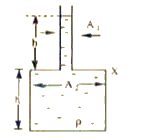Doubtnut App - Get 50 Lakh+ video solutions better than GoogleInstall Now

# The vessels shown in the figure has two sections of areas of cross - section A1andA2. A liquid of density ρ fills both the sections, up to a height h is each. Neglect atmospheric pressure.a) The pressure at the base of the vessel is 2hρg. b) The force exerted by the liquid on the base of the vessel is 2hρgA2. c) The weight of the liquid is <2hρgA2. d) The walls of the vessels at the level X exert a downward force hρg(A2−A1) on the liquid.

A

a, and c are correct

B

b, c and d are correct

C

a, c and d are correct

D

All are correct